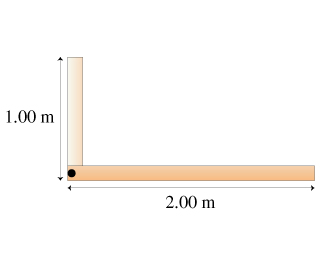# Problem: The figure shows two thin beams joined at right angles. The vertical beam is 15.0 kg and 1.00 m long and the horizontal beam is 25.0 kg and 2.00 m long.a. Find the center of gravity of the two joined beams. Express your answer in the form x,y, taking the origin at the corner where the beams join.b. Calculate the gravitational torque on the joined beams about an axis through the corner.

###### FREE Expert Solution

Center of gravity:

$\overline{){\mathbf{\left(}}{\mathbf{x}}{\mathbf{,}}{\mathbf{y}}{\mathbf{\right)}}{\mathbf{=}}{\mathbf{\left(}}\frac{{\mathbf{m}}_{\mathbf{1}}{\mathbf{x}}_{\mathbf{1}}\mathbf{+}{\mathbf{m}}_{\mathbf{2}}{\mathbf{x}}_{\mathbf{2}}}{{\mathbf{m}}_{\mathbf{1}}\mathbf{+}{\mathbf{m}}_{\mathbf{2}}}{\mathbf{,}}\frac{{\mathbf{m}}_{\mathbf{1}}{\mathbf{y}}_{\mathbf{1}}\mathbf{+}{\mathbf{m}}_{\mathbf{2}}{\mathbf{y}}_{\mathbf{2}}}{{\mathbf{m}}_{\mathbf{1}}\mathbf{+}{\mathbf{m}}_{\mathbf{2}}}{\mathbf{\right)}}}$

gravitational torque on the joined beams:

$\overline{){\mathbf{\tau }}{\mathbf{=}}{\mathbf{\left(}}{{\mathbf{m}}}_{{\mathbf{1}}}{\mathbf{g}}{\mathbf{\right)}}{{\mathbf{x}}}_{{\mathbf{1}}}{\mathbf{+}}{\mathbf{\left(}}{{\mathbf{m}}}_{{\mathbf{2}}}{\mathbf{g}}{\mathbf{\right)}}{{\mathbf{x}}}_{{\mathbf{2}}}}$

The torque due to gravity and about the corner ignores the y-component.

a)

Mass of horizontal beam, m1 = 25 kg

Mass of vertical beam, m2 = 15 kg

Length of horizontal beam, l1 = 2m

Lenght of vertical beam, l2 = 1m

Centre of gravity of horizintal beam, (x1, y1) = (l1/2, 0) = (1m, 0)

Centre of gravity of vertical beam, (x2, y2) = (0, l2/2) = (0, 0.5m)

100% (413 ratings)###### Problem Details

The figure shows two thin beams joined at right angles. The vertical beam is 15.0 kg and 1.00 m long and the horizontal beam is 25.0 kg and 2.00 m long.a. Find the center of gravity of the two joined beams. Express your answer in the form x,y, taking the origin at the corner where the beams join.

b. Calculate the gravitational torque on the joined beams about an axis through the corner.